Scan QR code or get instant email to install app

Question:

# 1212A cube with a surface area of 384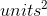is placed below a square pyramid with a height of 8 units and a side length of 3 units. A student wants to make a model of the combined object that is 25% smaller in volume than the object. What is the volume of the model?

A 1212402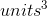explanation

1212The surface area of a cube is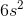, where s is the side length of the cube. So,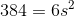gives 8 = s.
The volume of a cube is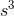, so the cube's volume is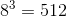.
The volume of a square pyramid is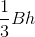, where B is the area of the square base and h is the height, so the pyramid's volume is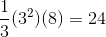.
The combined volume of the pyramid and cube is 512 + 24 = 536, and 25% smaller than this volume is the same as multiplying the volume by 0.75, which gives 536(0.75) = 402.